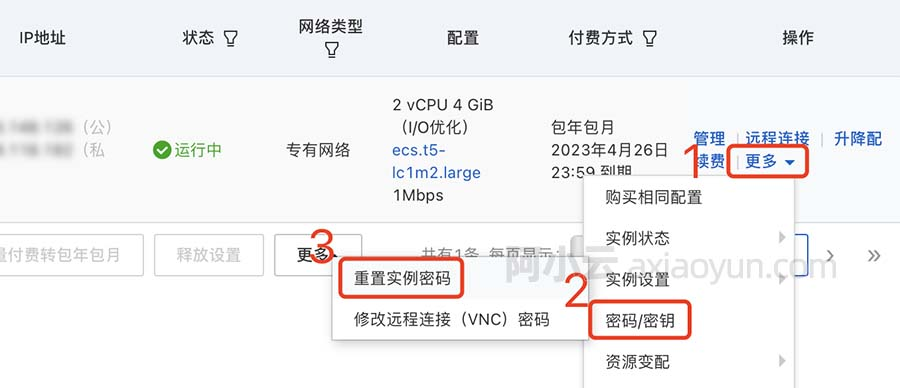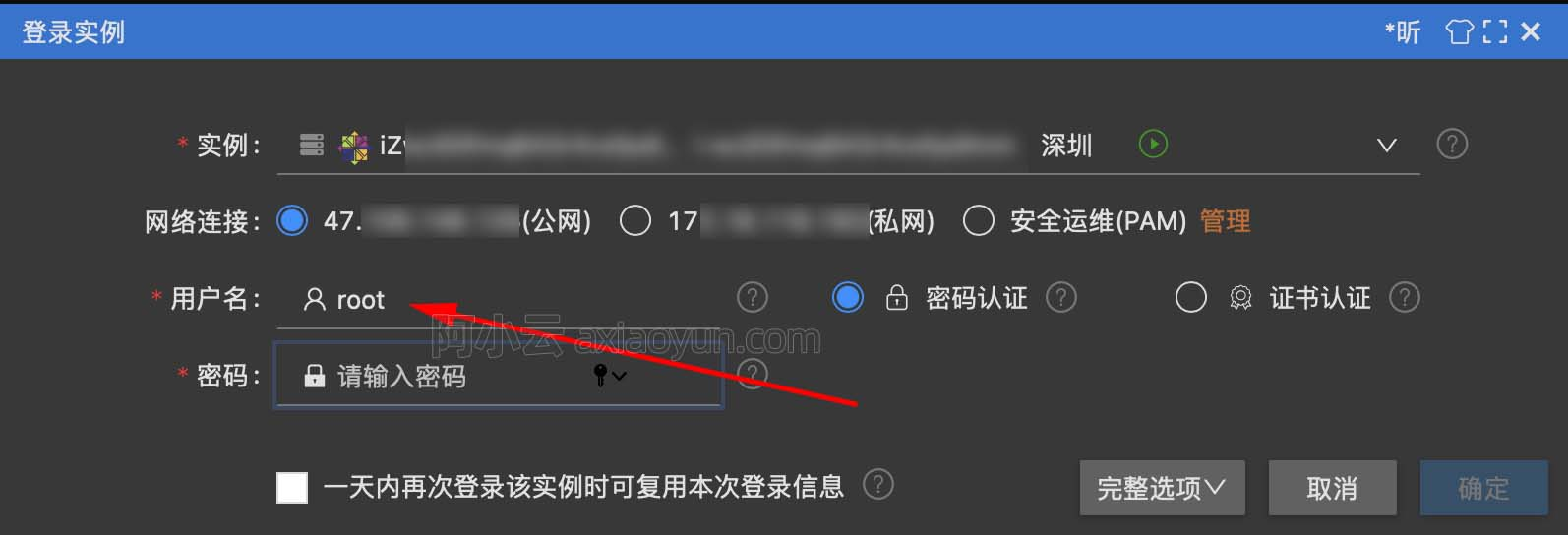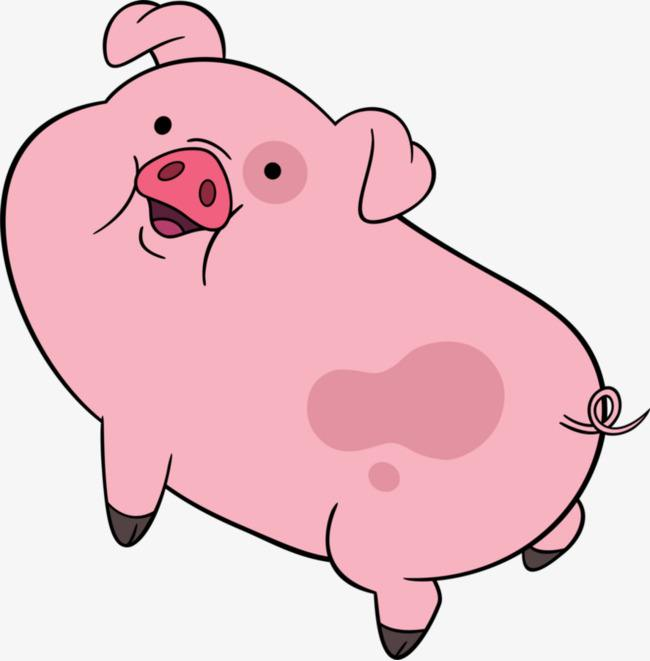Javascript中的prototype

+关注继续查看

prototype 是在IE4及其以后版本引入的一个针对于某一类的对象的方法，而且特殊的地方便在于：它可以给一个给类的对象添加方法。

1 最简单的例子，了解prototype：

② Boolean.rev(): 作用，布尔变量取反

2 已有方法的实现和增强，初识prototype：

(1) Array.push(new_element)

Array.prototype.push = function(new_element){
this[this.length]=new_element;
return this.length;
}

让我们进一步来增强他，让他可以一次增加多个元素！

Array.prototype.pushPro = function() {
var currentLength = this.length;
for (var i = 0; i < arguments.length; i++) {
this[currentLength + i] = arguments[i];
}
return this.length;
}

以此类推，你可以考虑一下如何通过增强 Array.pop 来实现删除任意位置，任意多个元素。

(2) String.length

实现方法：
String.prototype.cnLength = function(){
var arr=this.match(/[^\x00-\xff]/ig);
return this.length+(arr==null?0:arr.length);
}
这里用到了一些正则表达式的方法和全角字符的编码原理，由于属于另两个比较大的类别，本文不加说明，请参考相关材料。

3 新功能的实现，深入prototype：

(1) String.left()

String.prototype.left = function(num,mode){
if(!/\d+/.test(num))return(this);
var str = this.substr(0,num);
if(!mode) return str;
var n = str.Tlength() - str.length;
num = num - parseInt(n/2);
return this.substr(0,num);
}

(2) Date.DayDiff()

Date.prototype.DayDiff = function(cDate,mode){
try{
cDate.getYear();
}catch(e){
return(0);
}
var base =60*60*24*1000;
var result = Math.abs(this - cDate);
switch(mode){
case "y":
result/=base*365;
break;
case "m":
result/=base*365/12;
break;
case "w":
result/=base*7;
break;
default:
result/=base;
break;
}
return(Math.floor(result));
}

alert((new Date()).DayDiff((new Date(2002,0,1)))) -> 显示 329
alert((new Date()).DayDiff((new Date(2002,0,1)),"m")) -> 显示 10

(3) Number.fact()

Number.prototype.fact=function(){
var num = Math.floor(this);
if(num<0)return NaN;
if(num==0 || num==1)
return 1;
else
return (num*(num-1).fact());
}9894 02956 014442 011536 010876 02484 07445 054665 07272 04402 0
it徐胖子
IT徐胖子
733

0

《2021云上架构与运维峰会演讲合集》

《零基础CSS入门教程》

《零基础HTML入门教程》